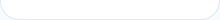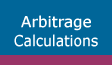Sports Arbitrage (Homepage)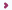Arbitrage CalculationsAdvanced Arbitrage Calculations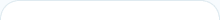After you have learned how to calculate arbitrage percentage, profit and individual bets for a simple two way arbitrage bet, we will explain you how to do the same calculations for a three way arb. In addition, we will show you how to calculate individual bets if you want certain winnings or if you have been limited at one of the bookmakers involved in the arbitrage situation.

## Three way arbitrage calculations

In order to get arbitrage percentage, profit and individual bets for a three way arbitrage you need to make the same calculations as for a two way arb. The only difference is that a three way arbitrage has three possible outcomes. These arbs are usually found in football matches where the possibility for a draw exists.

For example, three bookmakers have the following odds for football match between Barcelona and Real Madrid:

• Pinnacle Sports has odds of 2.1 for Barcelona to win
• Intertops has odds of 5.82 for Real Madrid to win
• 5Dimes has odds of 3.8 for a draw (tie)

Firstly, we calculate arbitrage percentages:

• 1/2.1 = 47.62%
• 1/5.82 = 17.18%
• 1/3.8 = 26.31%
total arbitrage percentage = 91.11%

Next, we calculate profit from this arbitrage bet:

(\$1000/91.11%) - \$1000 = \$97.57 or 9.76%

Finally, we calculate individual bets that need to be placed at three online bookmakers according to the total investment of \$1000:

• (\$1000*47.62%)/91.11% = \$522.66
• (\$1000*17.18%)/91.11% = \$188.57
• (\$1000*26.31%)/91.11% = \$288.77

## Different individual bets calculations

This section explains calculation of individual bets if you have been limited at one bookmaker involved in the arb and calculation if you want certain winnings. The basic calculation that is necessary to make all other calculations is to divide individual arbitrage percentages with total percentage. By doing this you actually calculate how much percent of the total investment amount you need to place on each outcome. We are still using the example from above.

• 47.62%/91.11% = 52.27%
• 17.18%/91.11% = 18.86%
• 26.31%/91.11% = 28.87%

Using these percentages you can calculate individual bets for certain total investment, winnings or you can calculate the remaining bet(s) when you have been limited by one bookmaker.

### Winnings of \$500 wanted

• 91.11% * \$500 = \$455.55
• 52.27% * \$455.55 = \$238.11
• 18.86% * \$455.55 = \$85.92
• 28.87% * \$455.55 = \$131.52

By multiplying total arbitrage percentage with wanted winnings we actually got total investment amount. After that it is easy to calculate individual bets. If you had placed \$238.11 on Barcelona to win at Pinnacle Sports, \$85.92 on Real Madrid to win at Intertops and \$131.52 on a draw at 5Dimes, you could have secured winnings from \$499.78 to \$500.05 and made surebet profit from \$44.23 to \$44.50.
Verification:
\$238.11 * 2.1 = \$500.03
\$85.92 * 5.82 = \$500.05
\$131.52 * 3.8 = \$499.78

### \$250 limit at 5Dimes with odds 3.8

• \$250 / 28.87% = \$865.95
• total investment = \$865.95
• 52.27% * \$865.95 = \$452.63
• 18.86% * \$865.95 = \$163.32

When you divide individual bet with percentage that bet makes up in total investment you get total investment amount. By using remaining two percentages you can figure out other individual bets. As you can see if you had placed \$452.63 at Pinnacle Sports on Barcelona to win, \$163.32 at Intertops on Real Madrid to win and \$250 at 5Dimes on a draw, you could have made profit from \$84.05 to \$84.57.
Verification:
\$452.63 * 2.1 = \$950.52
\$163.32 * 5.82 = \$950.52
\$250 * 3.8 = \$950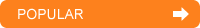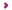Sports Arbitrage SoftwareArbitrage CalculatorRebelBetting - excellent arbitrage betting software that makes arbing simple even for complete beginners

Pinnacle Sports - best odds, highest limits; perfect bookie for arbitrage traders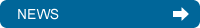New content!

Which currency to use for sports arbitrage?